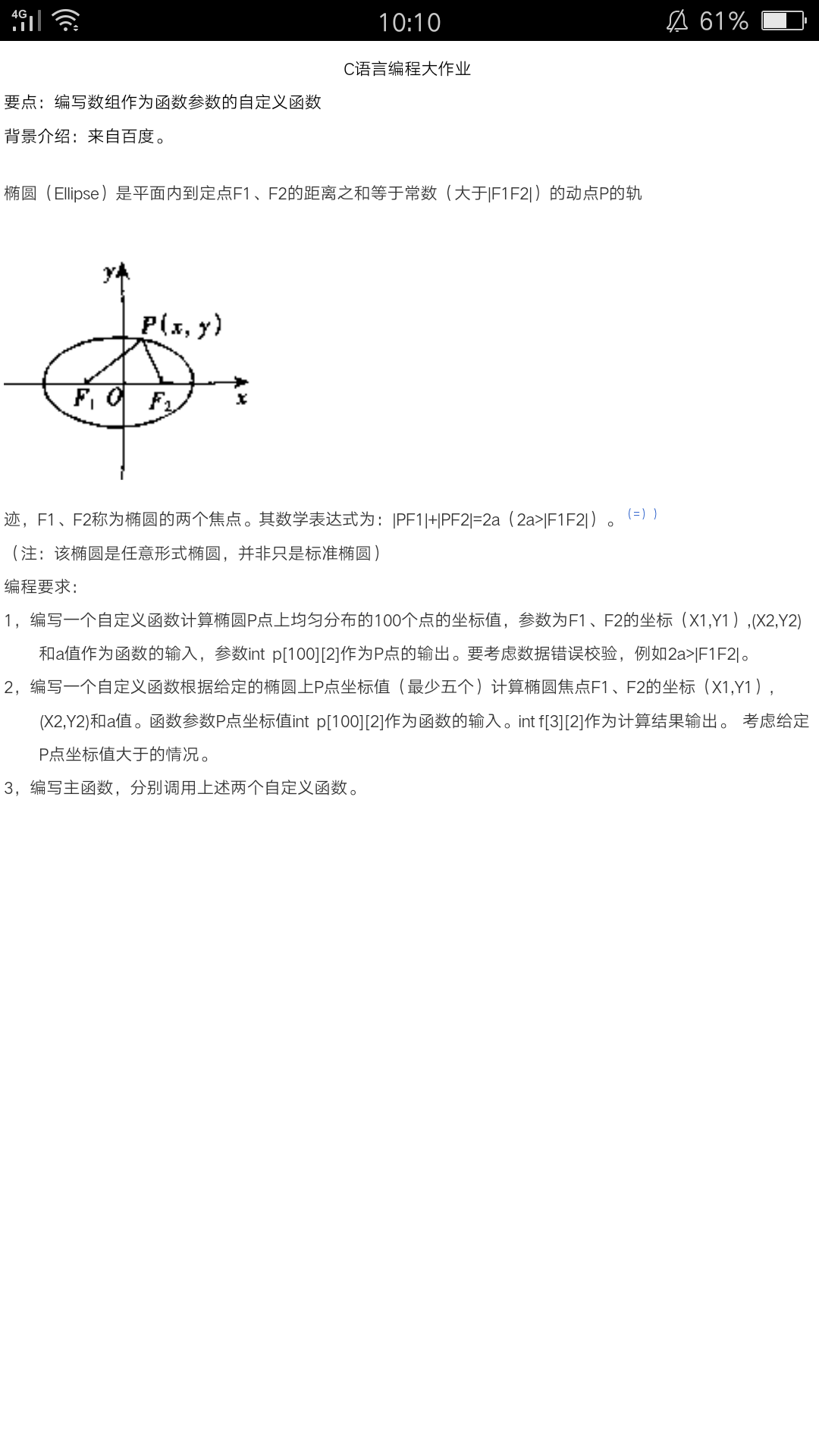1个回答

#include
#include
#include
void f(float x1,float x2,float y1,float y2,float a)
{
float f1f2,b,c,m,n,k,angle;
int i,j;
float p
f1f2=sqrt((x1-x2)*(x1-x2)+(y1-y2)*(y1-y2));
c=f1f2/2;
if(c>=a)
printf("输入的数据不合理");
else
{
b=sqrt(a*a-c*c);
k=(y2-y1)/(x2-x1);
angle=atan(k);
m=(x1+x2)/2;
n=(y1+y2)/2;
for(i=0;i<50;i++)
{
x=(-a)+(i+1)*2*a/51;
yz=b*sqrt(1-x*x/a/a);
yf=-yz;
p[i]=(x+m)*cos(angle)+(yz+n)*sin(angle);
p[i]=(yz+n)*cos(angle)-(x+m)*sin(angle);
p[i+50]=(x+m)*cos(angle)+(yf+n)*sin(angle);
p[i+50]=(yf+n)*cos(angle)-(x+m)*sin(angle);
}
for(j=0;j<100;j++)
printf("(x%d,y%d)=(%f,%f)",j+1,j+1,p[j],p[j];
}
}
int main(void)
{
float x1,x2,y1,y2,a;
int i;
printf("请输入椭圆的焦点：(x1,y1),(x2,y2)和a值:");
scanf("%f%f%f%f%f",&x1,&x2,&y1,&y2,&a);
f(x1,x2,y1,y2,a);
system("pause");
return 0;
}